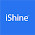### TDA 2030 audio Amplifier 20 Watt with Voltage divider Transistor Preamp Single Supply

The circuit was assembled on a good quality PCB and was tested on a 8-inch speaker load. The transistor pre-amp enhanced the gain and sound quality of the amplifier. If you want to make a low power amplifier for yourself try the above circuit. The parts used in this amplifier is easily available in the market. If you want to see the video tutorial click the link below :

### Watch Video Now ?

Hello friends, welcome to Electronics Tube Blogspot. In this blog we will be learning to make a 15 Watt Audio Amplifier with preamplifier to increase the gain and audio quality of the amplifier. The amplifier will be suitable to drive 4-inch to 6-inch woofer. The power supply used is single supply 0 12v 1 Ampere.
Now lets talk about the electronics components used in this audio amplifier. The amplifier is built using TDA2030 famous 5-pin audio IC . It has a max power dissipation of 20 Watts. The circuit diagram of this amplifier is provided in this blog. The voltage divider preamplifier ciruit is built using common General Purpose NPN transistors i.e BC547. The gain can of the preamplifier can be controlled via VR1 Potentiometer.

Components List :

Resistors :
R1=10K, R2=1.8K, R3=3.3K, R4=220ohm,
R5=R6=R7=100K, R8=4.7K, R9=150K, R10=1ohm, VR1=10K

Capacitors :
Cin=10uF, C1=0.47uF C2=22uF, C3=10uF, C4=2.2uF, C5=1000uF, C6=1000uF, C7=0.1uF.

Note: All capacitors except C7 are electrolytic. C7 is ceramic capacitor(104).
T1=BC547, D1 & D2 = 1n4007, IC=TDA2030

If you have any isuues please comment below !!!